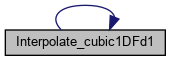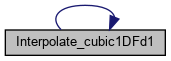FLASH-X Doxygen Generated Documentation From Interface Source Code
Interpolate_cubic1DFd1.F90 File Reference

Go to the source code of this file.

## Functions/Subroutines

real function, dimension(1:2) Interpolate_cubic1DFd1 (a, x)

## ◆ Interpolate_cubic1DFd1()

 real function, dimension (1:2) Interpolate_cubic1DFd1 ( real, dimension (1:4), intent(in) a, real, intent(in) x )

Calculates the function value and the rescaled 1st derivative value for a single [x] rescaled [0,1] coordinate and the 4 monocubic expansion coefficients. The monocubic expansion reads, for one line, in terms of the rescaled [0,1] x coordinate:

3 i F (x) = sum a (i) x i=0

The location index of the a (i) inside the 4-dimensional vector is:

location index of a (i) = 1 + i

The rescaled derivatives are given by the general formula:

r r 3 i-r d / dx = sum (i) * a (i) * x i=r r

where the Pochhammer symbols are defined as:

(i) = i * (i-1) * (i-2) * ... * (i-r+1) r

From the derivative formula we see, that the highest non-zero derivative is of 3-rd order.

a (i) : the i-th monocubic expansion coefficient x : rescaled [0,1] x coordinate

NOTES

1) The function is defined as a real array of size 2:

Interpolate_cubic1DFd1 (1) = the function value Interpolate_cubic1DFd1 (2) = the rescaled d/dx value

2) The code checks, if the supplied coordinate [x] is rescaled.

Definition at line 58 of file Interpolate_cubic1DFd1.F90.

References Interpolate_cubic1DFd1().

Referenced by Interpolate_cubic1DFd1().

Here is the call graph for this function:Here is the caller graph for this function: